#Quality Digest

Featured Product
This Week in Quality Digest Live
Statistics Features
Donald J. Wheeler
How you sample your process matters
Harish Jose
Using OC curves to generate reliability/confidence values
Donald J. Wheeler
The consequences of trying to fine-tune your charts
Donald J. Wheeler
The origin of the error function
Donald J. Wheeler
Using process behavior charts in a clinical setting
Statistics News
How to use Minitab statistical functions to improve business processes
New capability delivers deeper productivity insights to help manufacturers meet labor challenges
Day and a half workshop to learn, retain, and transfer GD&T knowledge across an organization
Elsmar Cove is a leading forum for quality and standards compliance
InfinityQS’ quality solutions have helped cold food and beverage manufacturers around the world optimize quality and safety
User friendly graphical user interface makes the R-based statistical engine easily accessible to anyone
Collect measurements, visual defect information, simple Go/No-Go situations from any online device
Good quality is adding an average of 11 percent to organizations’ revenue growth
Ability to subscribe with single-user minimum, floating license, and no long-term commitmentBio

Statistics

## When Zero Isn’t Zero: How to Handle Lower Detection Limits

### Calculate Ppk and control limits for processes with nondetects

Published: Monday, March 15, 2021 - 13:03

Traditional statistical methods for computing the process performance index (Ppk) and control limits for process-control purposes assume that measurements are available for all items or parts. If, however, the critical-to-quality (CTQ) characteristic is something undesirable, such as a trace impurity, trace contaminant, or pollutant, the instrument or gauge may have a lower detection limit (LDL) below which it cannot measure the characteristic. When this is the case, a measurement of zero or “not detected” does not mean zero; it means that the measurement is somewhere between zero and the LDL.

If the statistical distribution is known and is unlikely to be a normal (i.e., bell curve) distribution, we can nonetheless fit the distribution’s parameters by means of maximum likelihood estimation (MLE). This is how Statgraphics handles censored data, i.e., data sets for which all the measurements are not available, and the process can even be done with Microsoft Excel’s Solver feature. Goodness-of-fit tests can be performed to test the distributional fit, whereupon we can calculate the process performance index Ppk and set up a control chart for the characteristic in question.

### Data censoring

The topic of data censoring usually comes up in reliability studies, where a certain number of parts are subjected to a cyclic stress, or an amount of time of use. Suppose, for example, 100 parts are tested for 50,000 cycles, or 1,000 hours, but only 70 fail at the end of the test. We have the number of cycles, or amount of hours, for which each of the 70 items lasted, but we know only that the other 30 would have failed some time after the end of the test. This is known as Type I censoring. Type II censoring takes place when we decide to stop the test after a predetermined number of items have failed. Both of these are right-censoring, i.e., the failure times past the end of the test are unknown.

Left-censoring takes place when, if we measure a characteristic for 100 items, and get zero or “not detected” for 30 of them, we know only that the 30 measurements are somewhere between 0 and the lower detection limit.

### Example

100 data were simulated from a normal distribution with a mean of four and a standard deviation of 0.5. The units of measurement may be, for example, parts per million of an impurity or a contaminant. The distribution in this kind of application is unlikely to be normal, but the familiar normal distribution is probably best for illustration purposes. The lower detection limit is meanwhile 3.5.

The histogram of the available data, as generated by Statgraphics, appears in figure 1. It looks roughly like a bell curve, but the portion below 3.5 is not available.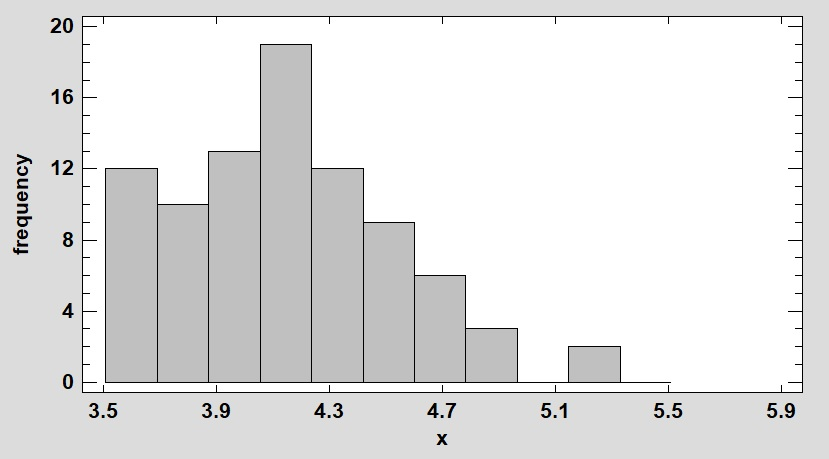Figure 1: Histogram of left-censored data

If, however, we present the data to Statgraphics in the format shown in figure 2, we can nonetheless estimate the mean and standard deviation from the information we have: 0 means the measurement is an actual measurement (not censored); –1 means it is left-censored and the indicated value is the lower detection limit; and +1 means it is right-censored, which does not apply in this example.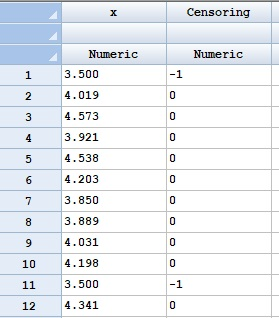Figure 2: Data entry format for censored data

If we then select from the Statgraphics menu bar Describe/Distribution Fitting/Fitting Censored Data, and fit the data to a normal distribution, Statgraphics estimates the mean at 4.03, and the standard deviation as 0.474. These results are reproducible in Excel by means of the Solver feature (an Excel add-in). You can download the Excel sheet that the following is based on.

### Maximum likelihood estimation (MLE)

MLE works by maximizing the natural log of the likelihood function, which is computed as follows for data set X, given parameter set Θ for the statistical distribution in question—in this case the mean and standard deviation of the normal distribution.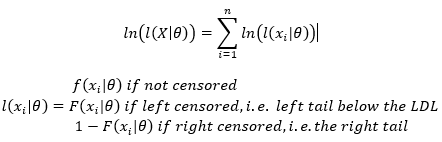where f is the probability density function, and F is the cumulative distribution. We can reproduce the Statgraphics results in Excel as follows. Set up the data as shown in figure 3.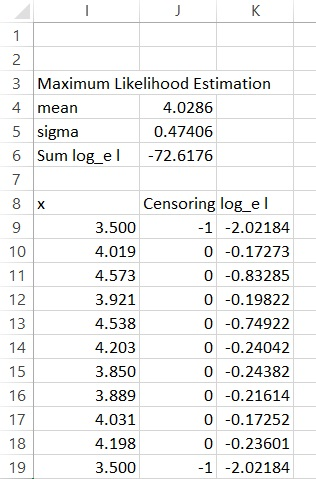Figure 3: Data organization for MLE optimization

The column log_e l contains the natural log of the likelihood function for each value of x. For the first row, 9, =IF(J9=0,LN(NORM.DIST(I9,mean,sigma,FALSE)),LN(NORM.DIST(I9,mean,sigma,TRUE))), which means that if cell J9 is zero and the number in I9 is an actual measurement, the probability density function of I9 given the indicated mean and sigma is used. Otherwise the cumulative distribution function of the lower detection limit is used. I have used a starting guess of three for the mean and 0.7 for the standard deviation, both of which are incorrect. Figure 4 shows how to use Excel’s Solver feature to maximize the log of the likelihood function, which is the sum of the 100 values in the column labeled log_e l.

1. The sum of the 100 individual log likelihood functions is in cell J6
2. The mean and standard deviation are in cells J4 and J5, which are named mean and sigma, respectively.
3. We can constrain the mean and standard deviations as shown; this might be a good idea to prevent the solution from straying from a realistic region. It also worked, however, without the constraints, so this is optional unless convergence on a solution fails.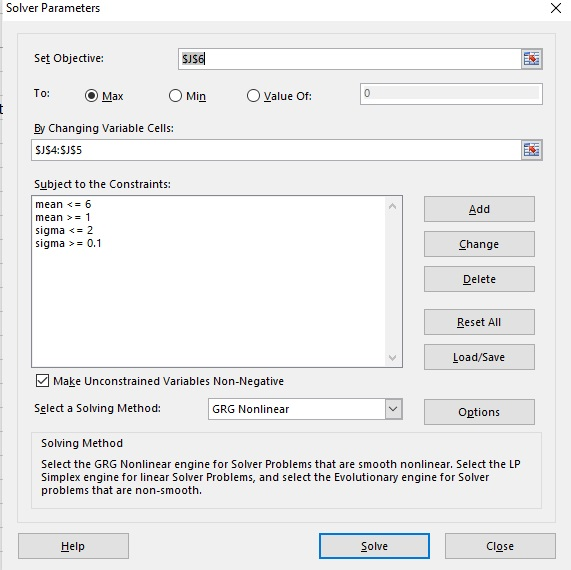Figure 4: Using Solver to maximize the likelihood function

Solver returns a mean of 4.03, a standard deviation of 0.474 (same as Statgraphics), and a new log likelihood function of –72.6 vs. the original –182.3. Figure 5, a Statgraphics X-Y-Z scatter plot of some log likelihood functions as computed in Excel, illustrates the basic concept. The goal is to adjust the parameters of the distribution, in this case the mean and standard deviation, to maximize the sum of the log likelihood functions.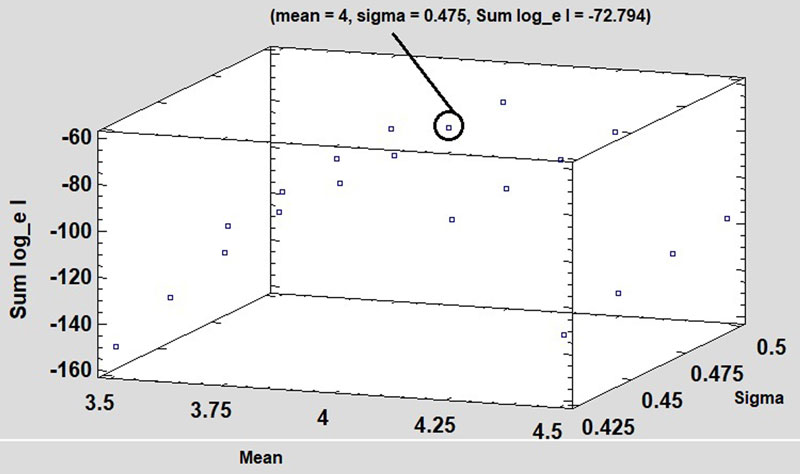Figure 5: Sum of log likelihood functions

### Caveats

The documentation for the Environmental Protection Agency’s free ProUCL software1, page 254, says, “The parametric maximum likelihood estimation (MLE) methods (e.g., Cohen 1991) and expectation maximization (EM) method (Gleit 1985) assume normality or lognormality of data sets and tend to work only when the data set has NDs with only one detection limit. These days, due to modern analytical tools and equipment, an environmental data set consists of NDs with multiple detection limits.” It adds that ProUCl 5.1 does not use MLE due to the difficulty in performing goodness-of-fit tests for applications with multiple detection limits.

• This article assumes we are dealing with a typical manufacturing or related process in which only one instrument, or type of instrument, is used, and it has a single LDL.
• MLE can be used successfully for other distributions, including the gamma distribution, and this is, in fact, an option in Statgraphics.

The reference also recommends against assuming that the nondetects are all halfway between zero and the lower detection limit (LDL/2). This could deliver rough estimates for the distribution parameters for starting guesses for iterative procedures, but that is about all.

### Tests for goodness of fit

It is always important to test the assumption that the data really do conform to the selected distribution, and Statgraphics offers subjective and quantitative tests for this. Figure 6 shows a quantile-quantile plot of the available data under the assumption that it follows a normal distribution. We want a good fit of the points to the regression line.Figure 6: Quantile-quantile plot

Statgraphics will also perform the chi square test for goodness of fit, in which the chi square test statistic has k-m-1 degrees of freedom when m parameters (in this case 2, the mean and standard deviation) are estimated from the data, and k histogram cells are used. The chi square statistic measures the “badness of fit” in terms of discrepancies between the observed and expected counts in each cell.where fj is the observed count, and Ej is the expected count in each cell. The latter should generally be five or more, and cells can be combined to make this happen.

The result, which depends on k, is shown in figure 7. The square root of the total number of data is often a good choice for the number of cells. The p value is the chance that the observed discrepancy between the observed cell counts and expected cell counts is due to random chance rather than non-normality, and p = 0.55 gives us no reason to reject this assumption. If we combine the last two cells to make the expected count more than five, then the contribution from that combined cell is ((10-8.71)^2)/8.71 = 0.19, which is not going to change the conclusion.Figure 7: Chi square test for goodness of fit using nine cells

### Regression on order statistics (ROS)

Regression on order statistics (ROS) is supported by the Environmental Protection Agency’s free ProUCL software, and it uses the mathematics of the quantile-quantile plot to obtain the parameters of the distribution. This works very well for the normal distribution, but it is more problematic for the gamma distribution, which requires the shape parameter (k or alpha) in order to set up the axis. If the distribution is normal, then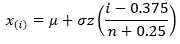where z is the standard normal deviate of the (i-0.375)/(n+0.25) quantile of the ith ordered measurement x(i). (i-0.5)/n also is sometimes used as the estimate of the quantile. The regression is performed, however, only on the observed measurements, while the nondetects are ignored. In this case, as there are 12 nondetects, the regression begins with the 13th ordered datum. Figure 8 illustrates the regression in Statgraphics. The intercept of 4.02 compares very favorably with the result (4.03) from maximum likelihood estimation, and the slope of 0.487 is comparable to 0.474, although not identical. A benefit of ROS is that this is a normal probability plot of the available measurements, and the correlation coefficient of 0.9970 indicates an excellent fit.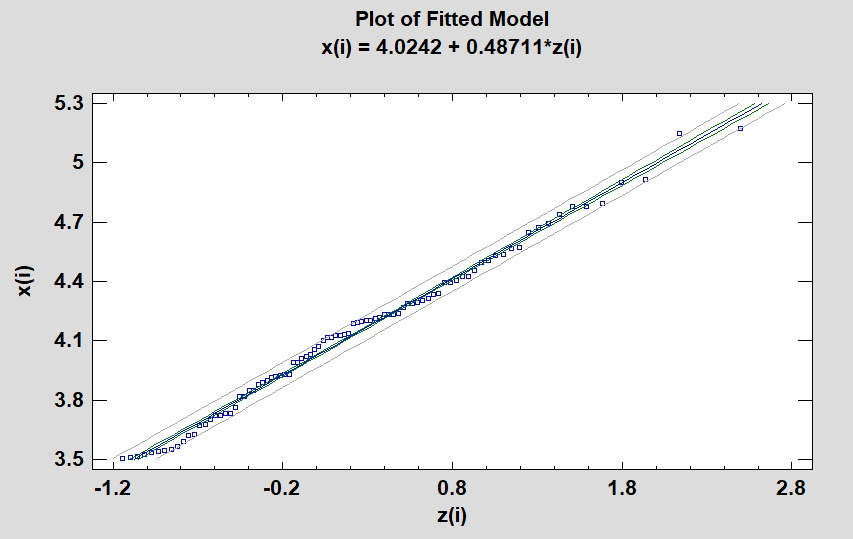Figure 8: Regression on order statistics (ROS) for left-censored normal data (normal probability plot)

### Deliverables: process performance index and SPC chart

Recall that the desired deliverables are the process performance index and control limits for an SPC chart. Both are straightforward, given the mean and standard deviation of the normal distribution. Suppose, for example, that the quality characteristic has an upper specification limit (USL) of 6.5; there is no lower specification limit because we do not care how little we get.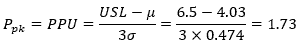This is better than a five-sigma process, i.e., one in which there are five process standard deviations between the mean and the specification limit, which means it is well able to meet the upper specification limit. The center line of the SPC chart for individual measurements is meanwhile the mean (4.03), and the upper control limit is the mean plus three standard deviations, or 5.45. The lower control limit is not only not relevant because we do not care how little of the undesirable quantity we get, but also because the LCL of 2.61 is below the lower detection limit.

Figure 9, from Statgraphics using “control to standard,” shows how the control chart might work in practice. It is understood that points at 3.5 are nondetects.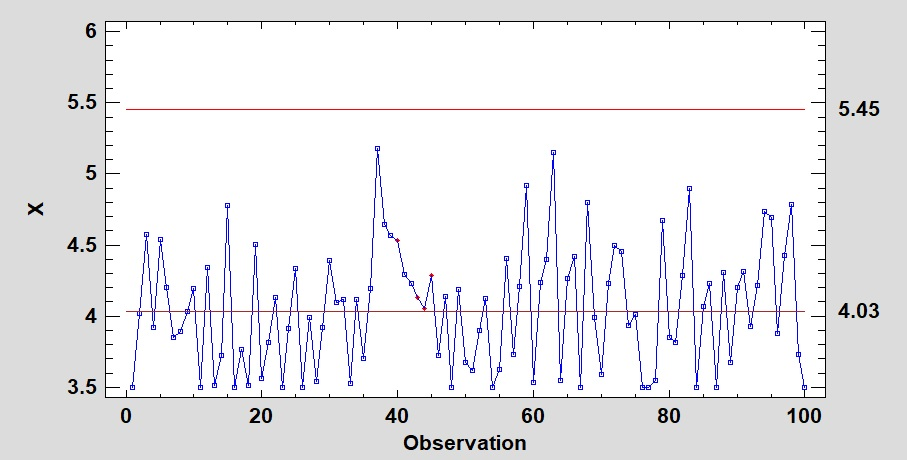Figure 9: Control chart for individuals.

### The gamma distribution

The gamma distribution is often a good model for undesirable random arrivals; the documentation for the EPA’s ProUCL software (version 5.1) says, “Many environmental data sets can be modeled by a gamma as well as a lognormal distribution.” Its probability function is as follows, where α (sometimes called k) is the shape parameter, and γ is the scale parameter. Excel uses 1/γ=ß for the scale parameter and includes a built-in function for this distribution.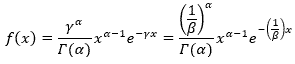100 measurements were simulated from a gamma distribution with shape parameter three and a scale parameter of six, for which the lower detection limit is 0.25 (e.g., parts per million of a contaminant or impurity). 17 measurements are nondetects, and Statgraphics finds α=3.506 and γ=7.21. Statgraphics’ quantile-quantile plot in figure 10 shows (subjectively) an excellent fit for the 83 observed measurements.Figure 10: Quantile-quantile plot, left-censored gamma distribution

Using Excel’s Solver feature (figure 11) delivers the same results (α=3.506, γ=7.214), where cell M5 is the sum of the log likelihood functions of each measurement. For row 8, =IF(M8=0,LN(GAMMA.DIST(L8,shape,1/scale,FALSE)),LN(GAMMA.DIST(L8,shape,1/scale,TRUE))), where GAMMA.DIST(L8,shape,1/scale,FALSE) is the probability density function of the measurement in cell L8, given the current shape and scale parameters, and where ß=1/γ, and GAMMA.DIST(L8,shape,1/scale,TRUE) is the cumulative density function of L8 if it is the lower detection limit, i.e., the measurement is left-censored.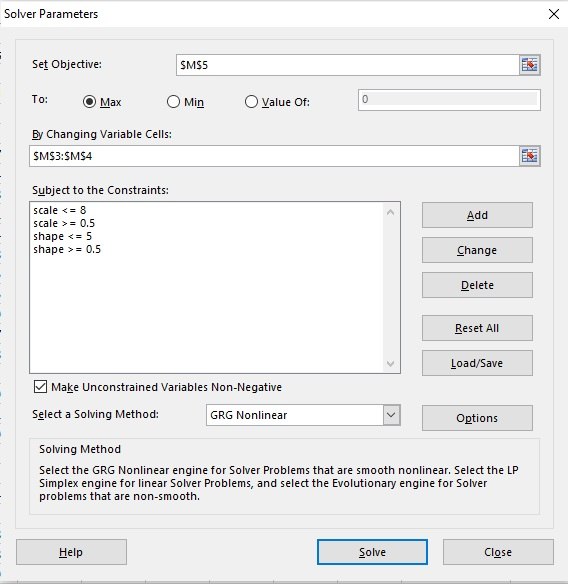Figure 11: Use of Solver on the gamma distribution

As it does for the normal distribution, Statgraphics will perform the chi square test for goodness of fit and offers other tests such as Kolmogorov-Smirnov.

Next, how do we obtain the desired deliverables if the upper specification is 1.5 ppm? In Excel,

=1-GAMMA.DIST(1.5,3.506,1/7.214,TRUE)

is the nonconforming fraction above the upper specification limit, and it is 0.00295. Statgraphics also can calculate these tail areas as shown in figure 12 or by using tables of critical values.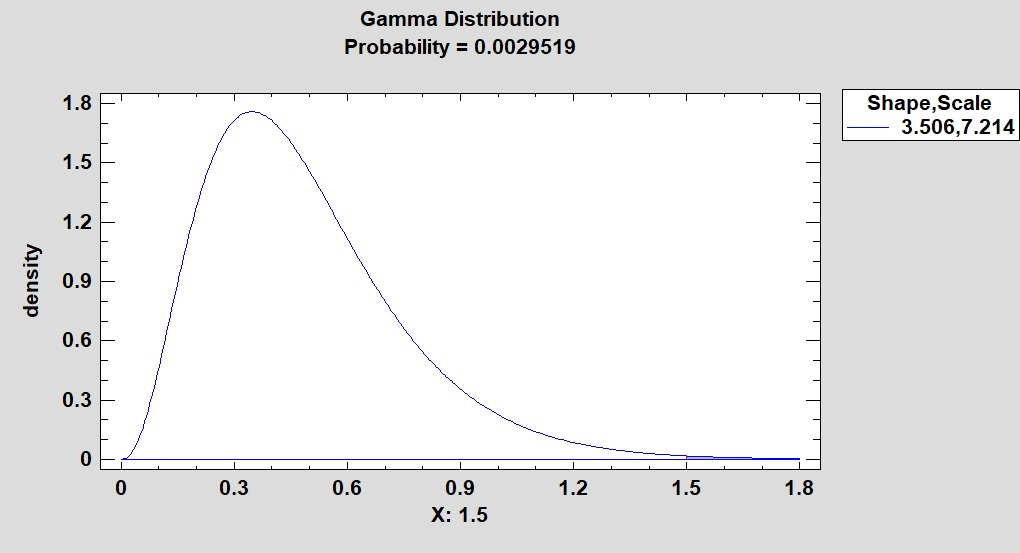Figure 12: Nonconforming fraction of a gamma distribution

### Deliverables for the gamma distribution

We can convert this into Ppk=PPU by converting this into the corresponding standard normal deviate. =NORMSINV(0.002952) =2.75 means that, for a normally distributed process, we would get a nonconforming fraction of 0.002952 for a specification limit 2.753 standard deviations from its mean. Divide by three to get Ppk=PPU=0.92, which means the process is not capable of meeting the upper specification limit.

The lower control limit for a control chart is unlikely to be relevant because we do not care how little of the undesirable quantity we get. The center line is the median of the gamma distribution, and its upper control limit is its 0.99865 quantile; this corresponds to the +3 sigma limit of a Shewhart control chart for a normal distribution.

In Excel, =GAMMA.INV(0.5,3.506,1/7.214) = 0.44 and =GAMMA.INV(0.99865,3.506,1/7.214)=1.636. These are the same limits we get in Statgraphics if we supply it with the indicated shape and scale parameters, and tell it to “control to standard” (i.e., use the known parameters), as shown in figure 13.Figure 13: SPC chart, gamma distribution

Statgraphics delivers the same results if, for the original data-fitting exercise, we ask for the critical values of the 0.00135, 0.5, and 0.99865 quantiles. The 0.00135 quantile of the lower control limit is presented only for comparison; it would not be used in practice because it is actually below the lower detection limit.

 Lower Tail Area (<=) Gamma 0.00135 0.045687 0.1 0.19688 0.5 0.44063 0.9 0.83399 0.99865 1.6358

The lower control limit =GAMMA.INV(0.00135,3.506,1/7.214) =  0.0457 can, however, be removed by setting the bottom of the ordinate to the lower detection limit of 0.25.

### Summary

When a manufacturing or related process has data with a known distribution and a single lower-detection limit, we can use maximum likelihood estimation to optimize the distribution’s parameters and then perform goodness-of-fit tests on the result. Once the parameters are in hand, we can:

1. Obtain the process performance index Ppk = PPU (as there is no lower specification limit) by calculating the nonconforming fraction above the upper specification limit, finding the corresponding standard normal deviate (z statistic), and dividing by three. The result is the PPU of a corresponding normally distributed process with the same nonconforming fraction.
2. Use the distribution’s median and 0.99865 quantile to set the center line and upper control limit for a control chart whose false-alarm risk of going over the UCL is the same as that of a Shewhart chart for a normally distributed process.

References
1. Singh, Anita, Ph.D., and Maichle, Robert. “ProUCL Version 5.1 User Guide; Statistical Software for Environmental Applications for Data Sets with and without Nondetect Observations,” Prepared for U.S. Environmental Protection Agency, 2015.

### Discuss### William A. Levinson

William A. Levinson, P.E., FASQ, CQE, CMQOE, is the principal of Levinson Productivity Systems P.C. and the author of the book The Expanded and Annotated My Life and Work: Henry Ford’s Universal Code for World-Class Success (Productivity Press, 2013).